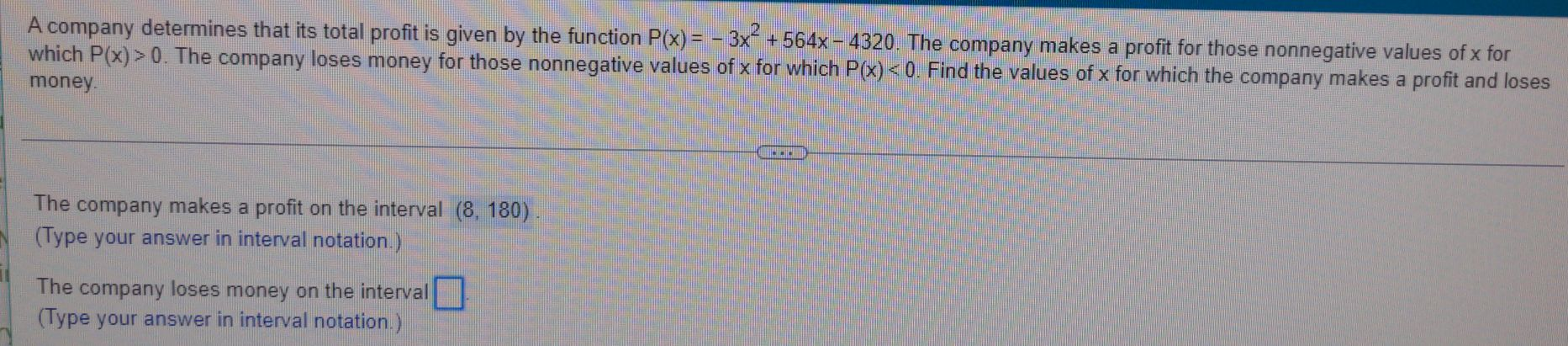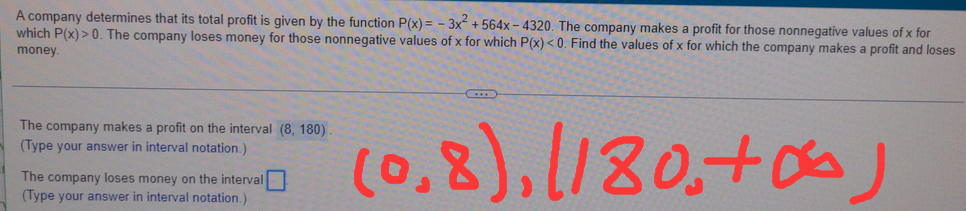### ¿Todavía tienes preguntas de matemáticas?

Pregunte a nuestros tutores expertos
Algebra
PreguntaA company determines that its total profit is given by the function $$P ( x ) = - 3 x ^ { 2 } + 564 x - 4320$$ . The company makes a profit for those nonnegative values of $$x$$ for which $$P ( x ) > 0$$ . The company loses money for those nonnegative values of $$x$$ for which $$P ( x ) < 0$$ . Find the values of $$x$$ for which the company makes a profit and loses money.

The company makes a profit on the interval $$( 8,180 )$$